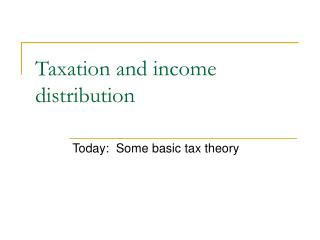DownloadDownload PresentationTaxation and income distribution

Taxation and income distribution

Télécharger la présentationTaxation and income distribution

- - - - - - - - - - - - - - - - - - - - - - - - - - - E N D - - - - - - - - - - - - - - - - - - - - - - - - - - -
Presentation Transcript

1. Taxation and income distribution Today: Some basic tax theory

2. Begin Unit 4 • Today • Parts of chapter 14 • An introduction to some basic theories related to taxation

3. Taxation • Taxes are typically used to finance public projects • Deadweight loss comes with most forms of taxation • This will be discussed in next lecture

4. Taxation and income distribution • The US federal tax system has been set up so that people with high incomes have higher average tax rates • Do people consume more leisure with high marginal tax rates? • Usually yes • Public project financing • People with high tax rates probably have high willingness to pay for many public projects

5. Taxation and income distribution Table 14.3 Average federal tax rates and share of federal taxes by income quintile (2006)

6. Some terminology • Statutory incidence • Who legally has to pay for the tax • Economic incidence • How much does real income change to all parties due to a tax?

7. Some terminology • Lump sum tax • A tax that has to be paid no matter how a person behaves • Proportional tax • Average tax rate is independent of income • Progressive tax • Average tax rate increases with income • Regressive tax • Average tax rate decreases with income

8. Some terminology • Unit tax • A tax that is paid per unit of a good • Ad valorem tax • A tax that is a percentage of the purchase price

9. Partial equilibrium models • With partial equilibrium models, only one market is examined at any one time • Ignores possible spillover effects • Usually easier to analyze than general equilibrium models • Two types of taxes analyzed • Unit tax • Ad valorem tax

10. Unit tax • You have likely seen unit taxes before • Econ 1 (or equivalent) • Econ 100A/B (or equivalent) • Either the buyer or seller pays a given dollar amount for each unit sold or purchased

11. \$ 0 Partial Equilibrium Models \$1.20 \$1.40 \$1.00 \$1.20 S1 S0 D1 D0 Quantity

12. Ad valorem taxes • Assume that the consumer pays an ad valorem tax • Example: 6% sales tax (of the net price) imposed on the consumer • The ad valorem tax shifts the demand curve by the same percentage (relative to the horizontal axis)

13. Ad valorem taxes Sf Price per pound of food Pr P0 Pm Df Df’ Qm Pounds of food per year Q0 Qr

14. Tax on gross price? …or net price? • A tax on the gross price (paid by consumers) lowers the willingness to pay by the percentage of the tax • Example • 25% tax of gross price when demand is P = 4000 – 20Q • New demand after tax is P = (1 – 0.25) (4000 – 20Q) • P = 3000 – 15Q

15. Tax on gross price? …or net price? • A tax on the net price (paid by consumers) is more complicated • You need to find the new demand such that when the tax is added, you get the new demand • Example • 25% tax of net price when demand is P = 4000 – 20Q • WTP with the tax is 5/4 of WTP without the tax • In other words, WTP without the tax is 4/5 the WTP with the tax • New demand after the tax is P = (4/5) (3000 – 20Q), which leads to P = 3200 – 16Q

16. Other types of taxes • Taxes from working • Income tax • Social Security tax • Hospital insurance tax (Medicare) • Capital taxes • Problem: Mobility of capital may move capital out of the country with taxation • Taxes on profits • Accounting profits • Economic profits

17. Summary • High income people pay a higher percentage of their income in taxes • Different forms of taxation exist • Unit tax • Ad valorem tax • Taxes on wages • Capital taxes • Profit taxes

18. Problem: Ad valorem tax • Supply: P = Q • Demand: P = 1710 – Q • What is the equilibrium in the absence of a tax? • What is the equilibrium if there is a 10% tax of the gross price?

19. No taxes • Set Q = 1710 – Q • Q = 855 • Since P = Q from the supply curve, P = 855

20. With a tax • A tax on the gross price implies that the willingness to pay goes down by 10% for each unit • New demand is P = 1539 – 0.9Q • Set Q = 1539 – 0.9Q • Q = 810

21. With a tax • What about price? • Price without the tax is 810 • This is the price that suppliers receive • Price with tax is 810/0.9 = 900 • This is the price that consumers pay

22. Inevitable: Death and…